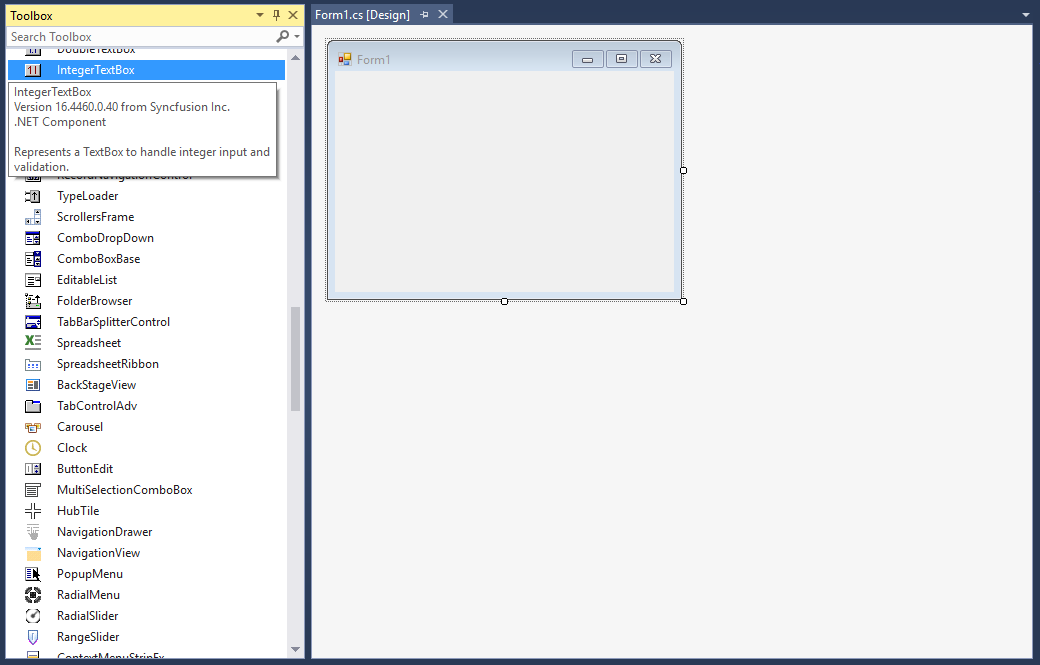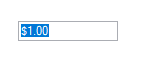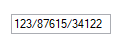# Getting Started with Windows Forms Integer TextBox

11 Oct 20222 minutes to read

## Assembly deployment

Refer to the control dependencies section to get the list of assemblies or NuGet package that needs to be added as a reference to use the control in any application.

You can find more details about installing the NuGet packages in a Windows Forms application in the following link:

How to install nuget packages

### Create a simple application with IntegerTextBox

You can create a Windows Forms application with IntegerTextBox using the following steps:

### Create a project

Create a new Windows Forms project in Visual Studio to display the IntegerTextBox control.

The IntegerTextBox control can be added to an application by dragging it from the toolbox to a designer view. The Syncfusion.Shared.Base assembly reference will be added automatically:## Add control manually in code

1. Add the Syncfusion.Shared.Base assembly reference to the project:

2. Include the Syncfusion.Windows.Forms.Tools namespace.

``using Syncfusion.Windows.Forms.Tools;``
``Imports Syncfusion.Windows.Forms.Tools``
3. Create an IntegerTextBox instance, and add it to the window.

``````IntegerTextBox integerTextBox1= new IntegerTextBox();
``````Dim integerTextBox1As IntegerTextBox = New IntegerTextBox()## Maximum and minimum value constraints

You can set the maximum and minimum values using the MaxValue and MinValue properties of IntegerTextBox.

``````this.integerTextBox1.MaxValue = 9223372036854775807;
this.integerTextBox1.MinValue = -9223372036854775808;``````
``````Me.integerTextBox1.MaxValue = 9223372036854775807
Me.integerTextBox1.MinValue = -9223372036854775808``````

## Number format

You can display the numbers in custom format using the NumberGroupSeparator and NumberGroupSizes properties of IntegerTextBox.

``````this.integerTextBox1.NumberGroupSeparator = "/";
integerTextBox1.IntegerValue = 1238761534122;
this.integerTextBox1.NumberGroupSizes = new int[] { 5 };``````
``````Me.integerTextBox1.NumberGroupSeparator = "/"
integerTextBox1.IntegerValue = 1238761534122
Me.integerTextBox1.NumberGroupSizes = New Integer() { 5 }``````